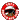Broadcast Sheet
 INDEX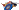Line 1 Basic CarLine 2 Basic CarLine 3 Build CodesLine 4 Build CodesLine 5 Build CodesLine 6 Sales CodesLine 7 Sales CodesLine 8 Sales CodesLine 9 Sales CodesLine 10 Sales CodesLine 11 Sales CodesLine Remarks'71 Charger Super Bee
 CONTACT INFORMATION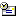John M. Bōber  Viera, FL 32955Chrysler Corporation Car Production Broadcast Sheet

Line 3 Build Codes.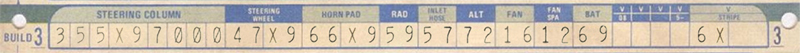Build codes run from line 3, 4 and 5. Most of the numbers are the last few digits of the "factory production" part numbers.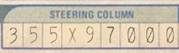Steering Column: The first three digits are the column type: 315 = B-body 4-speed 351 = B-body Column shift Auto Trans 355 = B-body Floor shift Auto Trans The next two digits are the column color: B5 = Blue F8 = Green X9 = Black The last four digits are the type of steering: 1000 = manual 1005 = manual A01 light group 7000 = non tilt power steering 7005 = non tilt power steering A01 light group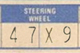Steering Wheel: The first two digits are the last two digits of the part number of the wheel: 37 = Tuff Wheel 47 = Simulated Wood Wheel The next two digits are the color: B5 = Blue X9 = Black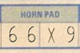Horn Pad: The first two digits are the last two digits of the part number of the horn pad: 19 = Tuff Wheel pad 66 = Simulated Wood horn ring The next two digits are the color: B5 = Blue X9 = Black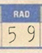RAD (Radiator): The two digits are the last two digits of the part number of the radiator: 61 = 3443961 22" 383 auto 62 = 3443961 22" 383 manual 38 = 3443938 22" 383/440 40 = 3443940 26" 383 A/C 59 = 3443959 26" 383/440/HEMI 60 = 3443960 26" 383/440 A/C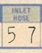Inlet Hose: The two digits are the last two digits of the part number of the hose: 14 = 3462114 57 = 2863257 86 = 3462186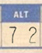Alt (Alternator): The two digits are the last two digits of the part number of the alternator: 10 = 2642610 60amp Single 28 = 2642228 60amp Single 29 = 2642229 60amp Dual 71 = 3438171 30amp Single 72 = 3438172 37amp Single 73 = 3438173 50amp Single 74 = 3438174 60amp Single 76 = 3438176 37amp Dual 77 = 3438177 50amp Dual 78 = 3438178 50amp Dual 78 = 3438378 34amp Single 79 = 3438179 60amp Dual 80 = 3438180 60amp Dual 80 = 3438780 37amp Single 82 = 3438782 50amp Single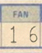Fan: The two digits are the last two digits of the part number of the fan: 16 = 2863216 [7 Blades]Fan Spacer: The two digits are the last two digits of the part number of the fan spacer or torque drive clutch unit of the fan: SPACERS 10 = 2120510 1.60 19 = 2863219 1.86 30 = 2120130 2.38 32 = 1825932 1.24 36 = 2265036 2.20 37 = 2265037 .90 46 = 1674746 1.72 55 = 1730155 1.40 56 = 1825956 2.00 59 = 1851959 1.06 60 = 1851960 .70 61 = 1851961 1.30 62 = 3462162 1.93 DRIVES 05 = Thermal 3462105 06 = Thermal 3462106 07 = Thermal 3462107 08 = Thermal 3462108 12 = Torque 3462112 14 = Torque 3769614 16 = Thermal 3769616 17 = Thermal 3769617 18 = Torque 3769618 23 = Thermal 3769623 33 = Torque 2863233 59 = Torque 2863259 60 = Thermal 2863260 61 = Thermal 2863261 63 = Thermal 2863263 64 = Thermal 2863264 66 = Thermal 2863266 67 = Thermal 2863267 68 = Torque 3462168 70 = Torque 2806070 74 = Thermal 3462174 79 = Thermal 3462179 80 = Torque 3462180 82 = Torque 3462182 83 = Torque 3462183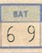Batteries: The two digits are the last two digits of the factory part number of the battery installed: 20 = 59 amp 51 = 46 amp 64 = 70 amp 66 = 55 amp 67 = 70 amp 69 = 70 amp 81 = 80 amp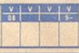V Codes: First box V0. If it has a 8 in the box it is for "delete" vinyl roof. The next two boxes are for V2_ codes, V3_ codes or V4_ codes V21 = Performance hood paint V22 = Delete hood paint V23 = Delete lower body color V24 = Engine ci Call outs V20 = Halo Vinyl top Convertible Top V3W = White V3X = Black V39 = Special Order Vinyl Canopy Roof V4W = White V4X = Black V4F = Green V4Y = Gold The last box V5_ is for Body side molding color: V50 = Chrome V5B = Blue V5E = Red V5F = Green V5G = Green V5R = Red V5W = White V5X = Black V5Y = Gold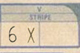V Stripe Codes: A two digit code:(Note: R/T's and Super Bee's only could have "BLACK" coloror stripe delete.) V6X = Black longitudinal stripes V68 = Stripe Delete Other Stripe codes (Add color code for *) V4* = Body Stripe V7* = Accent Stripe V8* = Transverse Stripe V9* = Sport stripeSponsors Ads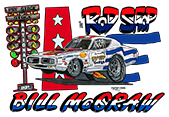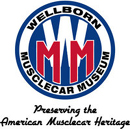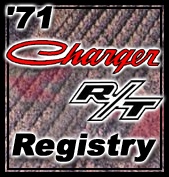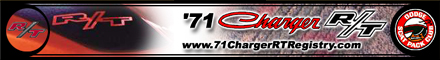71 Super Bee

"Mopar® is a registered trademark of Chrysler LLC"